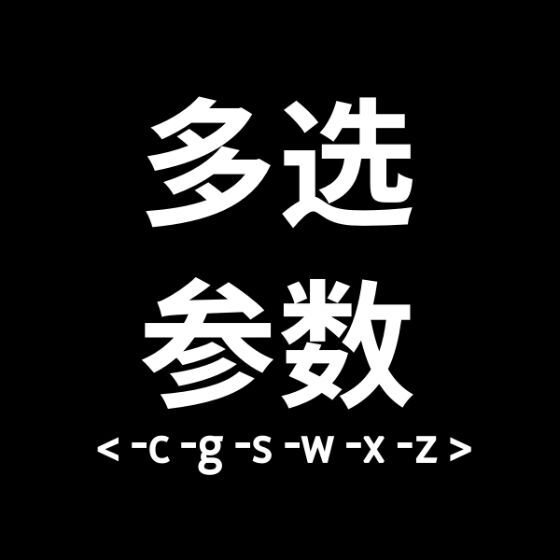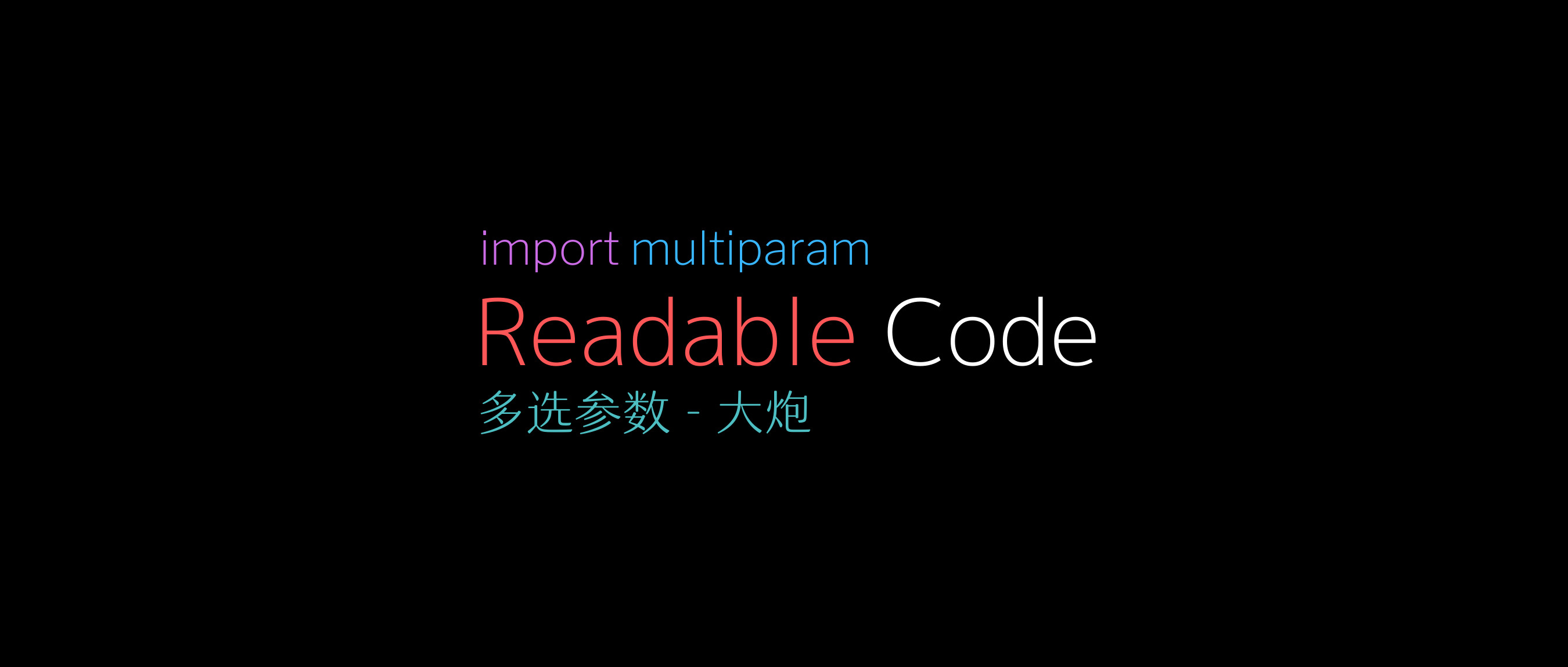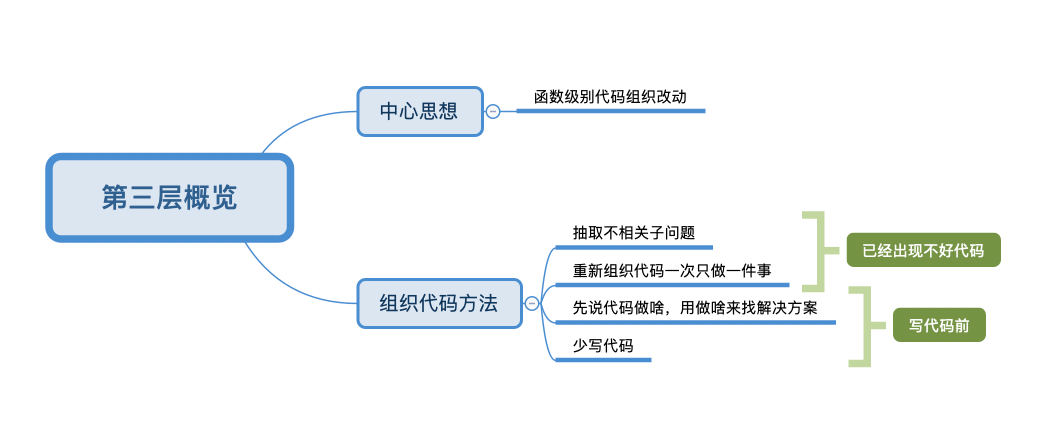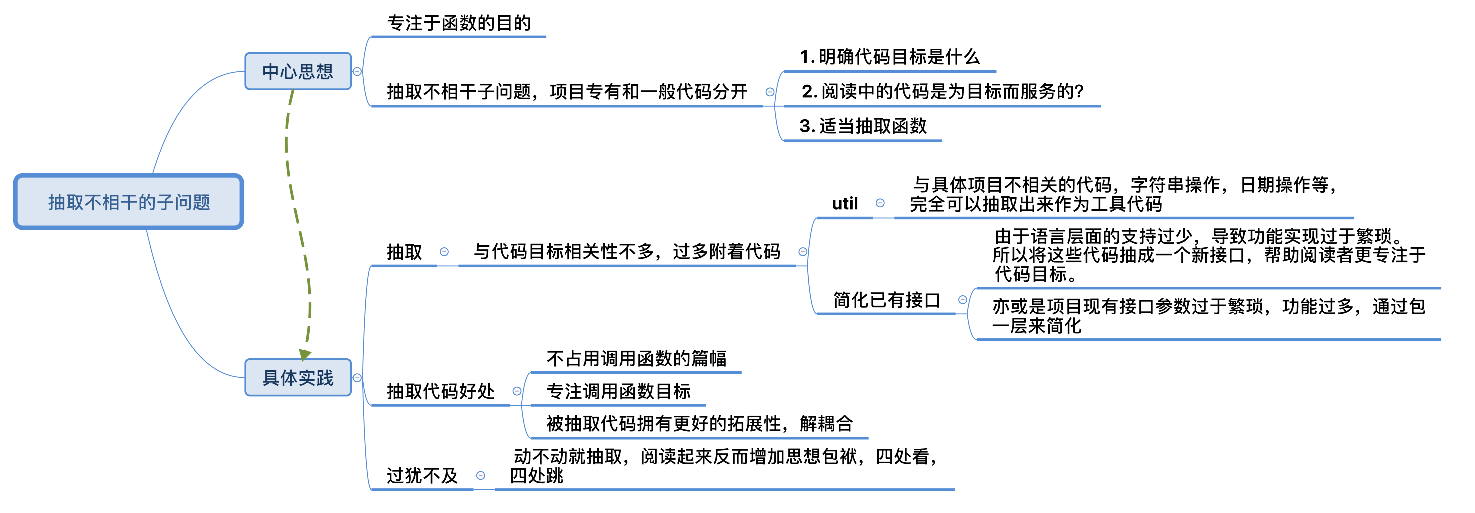# 可读代码编写炸鸡九 - 抽取子问题• 当你面对已经出现的不好代码该咋办

• 在你写代码前如何尽量避免不好代码# 写在前头1. 看一下整个代码块或者一个函数，问一下自己，这个代码的 最终目的 是什么？

2. 对于代码的每一行，问一下自己，这样对这个目标有 直接作用 吗。还是这代码用于解决其他的不相关的子问题

3. 如果解决不相关的子问题的代码开始变多，问一下自己，是不是需要提取，封装为函数

# 芜湖，如何抽取

这个代码的最终目的是什么

function wananDoA()    -- do something about a    ...    -- do something about b    ...    ...    ...    ...    ...    -- do something about c    ...    ...    ...    ...    ...        -- do something about a    ...end

function wananDoB()    -- do something about b    ...    ...    ...    ...    ...endfunction wananDoC()    -- do something about c    ...    ...    ...    ...    ...endfunction wananDoA()    -- do something about a    ...    wananDoB()    wananDoC()    -- do something about a    ...end

• 不占用调用函数的篇幅

• 专注调用函数目标

• 被抽取代码拥有更好的拓展性，解耦合

## 工具代码

function onDayPass()    -- 获取玩家数据    ...    ...        -- 结算    ...    ...    -- 数据落地    ...    ...end

function onDayPass()    -- 获取玩家数据    local user = getUser()    ...    ...        -- 结算    ...        -- 指定区间        local beg, ending = readActiveIntervalConfig()    local curTime = os.time()    if curTime > ending then return false end    if curTime < beg then return false end            -- 上下线时间是否同一天    local elapse = user.logoutTime - user.loginTime >= 0 and user.logoutTime - user.loginTime or user.loginTime - user.logoutTime    if elapse > 86400 then        return false    end    if os_date("%j",user.logoutTime ) != os_date("%j",user.loginTime) then        return false    end    ...    ...    -- 数据落地    ...    ...end

-- 当前时间是否在一个区间内function isInInterval(beg, ending)    local curTime = os.time()    if curTime > ending then return false end    if curTime < beg then return false endend-- 绝对值function mathAbs(t1, t2)    return t1 - t2 >= 0 and t1 - t2 or t2 - t1end-- 是否在同一天function isSameDay(t1, t2)    if mathAbs(t1, t2) > 86400 then        return false    end        if os_date("%j",t1 ) != os_date("%j", t2) then        return false    end        return trueend

function onDayPass()    -- 获取玩家数据    local user = getUser()    ...    ...        -- 结算    ...    -- 指定区间        local beg, ending = readActiveIntervalConfig()    if not isInInterval(beg, ending) then        return false    end        -- 上下线时间是否同一天    if not isSameDay() then        return false    end    ...    ...    -- 数据落地    ...    ...end

## 简化已有接口

for key, _ in pairs(t) do    t.key = nilend

table.clear = function(t)    for key, _ in pairs(t) do        t.key = nil    endend

function interface(uid, aid, params, isSave, ...)    ...    ...    ...end

function saveInterfaceWithRewardParams(uid, aid, ...)    local params = {        id     = 1001,        type   = 1,        amount = 3,    }    return interface(uid, aid, params, true, ...)end

# 不要过分抽取

function _doA()    ...endfunction _doB()    ...endfunction _doC()    ...endfunction _doD()    ...endfunction _doE()    ...endfunction wannaDoA()    _doA()    _doB()    _doC()    _doD()    _doE()end

# 总结

1. 阅读者注意力。

2. 函数的目的。

3. 篇幅。## 评论